# XLOOKUP – Multiple Sheets at Once – Excel & Google Sheets

This tutorial will demonstrate how to perform a XLOOKUP on multiple sheets in Excel. If your version of Excel does not support XLOOKUP (or you are using Google Sheets), read how to use the VLOOKUP instead.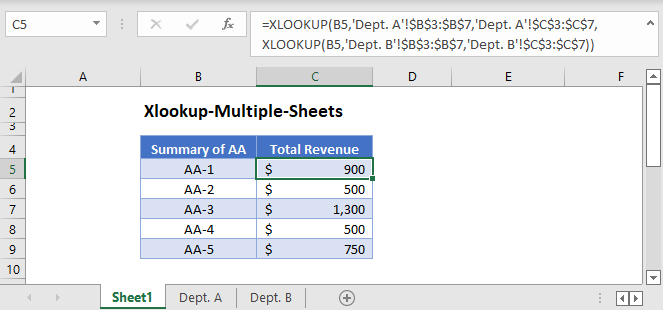The XLOOKUP Function can only perform one lookup per set of data. If we want to perform a lookup among multiple sets of data that are stored in different sheets, we can nest another XLOOKUP in the 4th argument (i.e., if_not_found) of the XLOOKUP Function.

The XLOOKUP Function (or other lookup formulas) returns the #N/A error if it can’t find a match, and we can use its 4th argument to replace the error with a customized value.

Instead of a customized value, we’ll nest another XLOOKUP to perform another lookup from another sheet if the first lookup can’t find a match in the first sheet.

### XLOOKUP – 2 Sheets at Once

``=XLOOKUP(B3,'Dept. A'!\$B\$3:\$B\$7,'Dept. A'!\$C\$3:\$C\$7,XLOOKUP(B3,'Dept. B'!\$B\$3:\$B\$7,'Dept. B'!\$C\$3:\$C\$7))``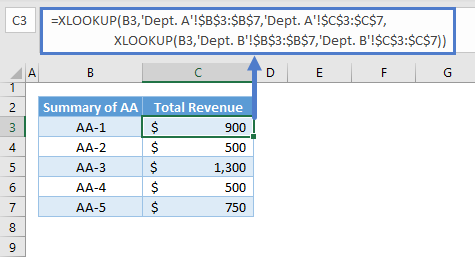Let’s breakdown and visualize the formula:

#### XLOOKUP Function

Here’s the XLOOKUP for the 1st sheet if the 4th argument is left empty:

``=XLOOKUP(B3,'Dept. A'!\$B\$3:\$B\$7,'Dept. A'!\$C\$3:\$C\$7)``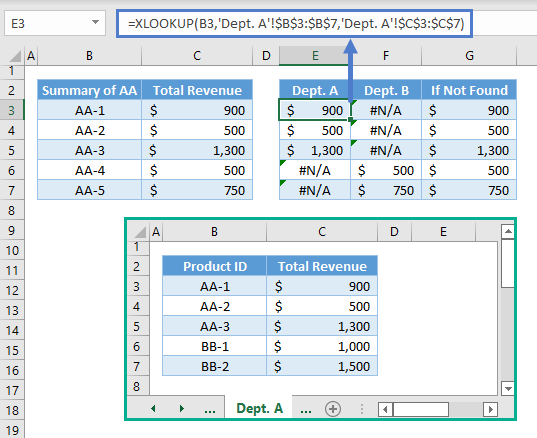Note: The XLOOKUP Function requires at least three arguments: lookup value, lookup array and return array. By default, the XLOOKUP Function finds an exact match from the top of the lookup array going down (i.e., top-down). Once it finds a match, it returns the corresponding value from the return array. Otherwise, it returns an error.

Here’s the XLOOKUP for the 2nd Sheet:

``=XLOOKUP(B3,'Dept. B'!\$B\$3:\$B\$7,'Dept. B'!\$C\$3:\$C\$7)``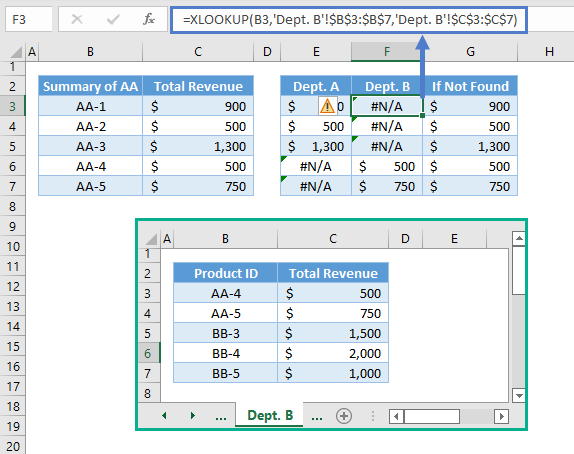The 4th argument of the XLOOKUP Function and the IFNA Function work the same way. They check if a value is the #N/A Error, and if it’s true, they will return the customized value that was set.

The result of the XLOOKUP for the 2nd sheet (e.g., Department B) are used as replacement values for the #N/A Errors from the lookup for the 1st sheet (e.g., Department A).

``=XLOOKUP(B3,'Dept. A'!\$B\$3:\$B\$7,'Dept. A'!\$C\$3:\$C\$7,F3)``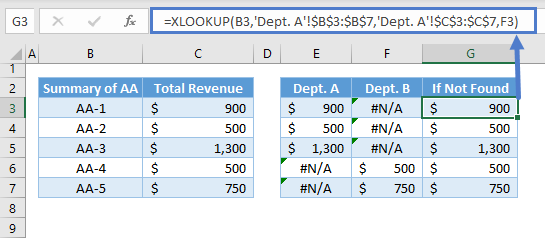Combining all these concepts results to our original formula:

``=XLOOKUP(B3,'Department A'!\$B\$3:\$B\$7,'Department A'!\$C\$3:\$C\$7,XLOOKUP(B3,'Department B'!\$B\$3:\$B\$7,'Department B'!\$C\$3:\$C\$7))``

### XLOOKUP – More than 2 Sheets at Once

To add more sheets, we just need to nest another XLOOKUP to the last XLOOKUP in the formula. Here’s the formula for 3 sheets:

``=XLOOKUP(B3,'Dept. A'!\$B\$3:\$B\$7,'Dept. A'!\$C\$3:\$C\$7,XLOOKUP(B3,'Dept. B'!\$B\$3:\$B\$7,'Dept. B'!\$C\$3:\$C\$7,XLOOKUP(B3,'Dept. C'!\$B\$3:\$B\$7,'Dept. C'!\$C\$3:\$C\$7)))``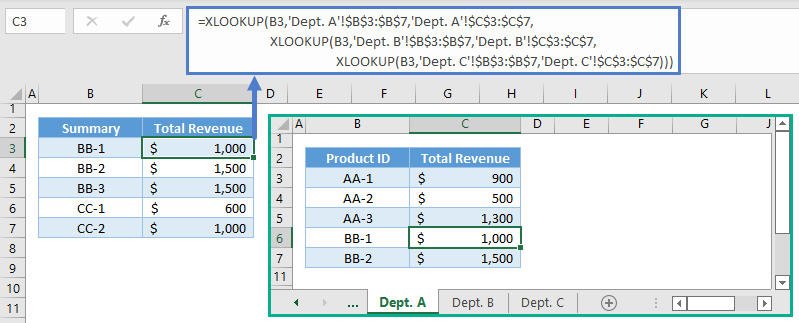### Excel Practice Worksheet

Practice Excel functions and formulas with our 100% free practice worksheets!